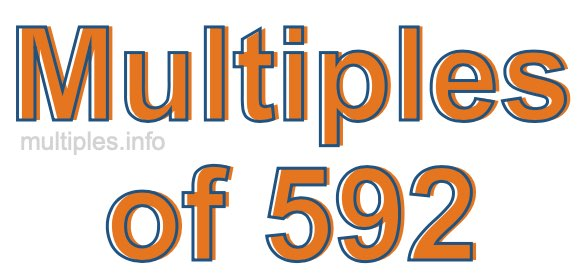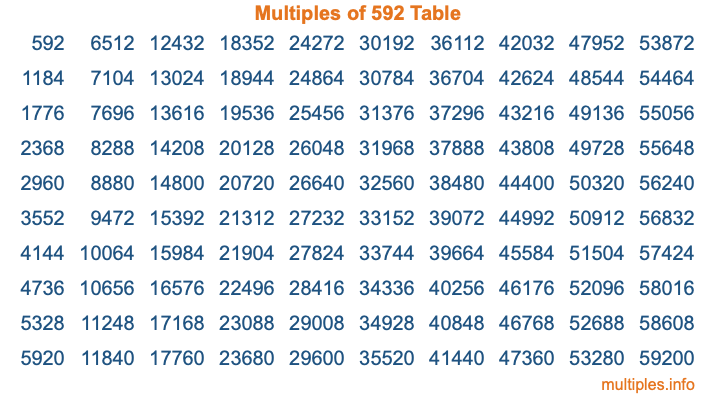Multiples of 592Welcome to the Multiples of 592 page. Here we will first teach you everything you will ever need to know about the multiples of 592, and then give you a study guide summary of everything we taught you to make sure you remember it all. Use this page to look up facts and learn information about the multiples of 592. This page will make you a multiples of five hundred ninety-two expert!

Definition of Multiples of 592
Multiples of 592 are all the numbers that when divided by 592 equal an integer. Each of the multiples of 592 are called a multiple. A multiple of 592 is created by multiplying 592 by an integer.

Therefore, to create a list of multiples of 592, you start with 1 multiplied by 592, then 2 multiplied by 592, then 3 multiplied by 592, and so on for as long as you want. Thus, the list of the first five multiples of 592 is 592, 1184, 1776, 2368, and 2960. To see a larger list of multiples of 592, see the printable image of Multiples of 592 further down on this page. We also have a category where you can choose any nth multiple of 592.

Multiples of 592 Checker
The Multiples of 592 Checker below checks to see if any number of your choice is a multiple of 592. In other words, it checks to see if there is any number (integer) that when multiplied by 592 will equal your number. To do that, we divide your number by 592. If the the quotient is an integer, then your number is a multiple of 592.

Is  a multiple of 592?

Least Common Multiple of 592 and ...
A Least Common Multiple (LCM) is the lowest multiple that two or more numbers have in common. This is also called the smallest common multiple or lowest common multiple and is useful to know when you are adding our subtracting fractions. Enter one or more numbers below (592 is already entered) to find the LCM.

Check out our LCM Calculator if you need more details about the Least Common Multiple or if you need the LCM for different numbers for adding and subtraction fractions.

nth Multiple of 592
As we stated above, 592 is the first multiple of 592, 1184 is the second multiple of 592, 1776 is the third multiple of 592, and so on. Enter a number below to find the nth multiple of 592.

th multiple of 592

Multiples of 592 vs Factors of 592
592 is a multiple of 592 and a factor of 592, but that is where the similarities end. All postive multiples of 592 are 592 or greater than 592. All positive factors of 592 are 592 or less than 592.

Below is the beginning list of multiples of 592 and the factors of 592 so you can compare:

Multiples of 592: 592, 1184, 1776, 2368, 2960, etc.

Factors of 592: 1, 2, 4, 8, 16, 37, 74, 148, 296, 592

As you can see, the multiples of 592 are all the numbers that you can divide by 592 to get a whole number. The factors of 592, on the other hand, are all the whole numbers that you can multiply by another whole number to get 592.

It's also interesting to note that if a number (x) is a factor of 592, then 592 will also be a multiple of that number (x).

Multiples of 592 vs Divisors of 592
The divisors of 592 are all the integers that 592 can be divided by evenly. Below is a list of the divisors of 592.

Divisors of 592: 1, 2, 4, 8, 16, 37, 74, 148, 296, 592

The interesting thing to note here is that if you take any multiple of 592 and divide it by a divisor of 592, you will see that the quotient is an integer.

Multiples of 592 Table
Below is an image of the first 100 multiples of 592 in a table. The table is in chronological order, column by column. The first column has the first ten multiples of 592, the second column has the next ten multiples of 592, and so on.The Multiples of 592 Table is also referred to as the 592 Times Table or Times Table of 592. You are welcome to print out our table for your studies.

Negative Multiples of 592
Although not often discussed or needed in math, it is worth mentioning that you can make a list of negative multiples of 592 by multiplying 592 by -1, then by -2, then by -3, and so on, to get the following list of negative multiples of 592:

-592, -1184, -1776, -2368, -2960, etc.

Multiples of 592 Summary
Below is a summary of important Multiples of 592 facts that we have discussed on this page. To retain the knowledge on this page, we recommend that you read through the summary and explain to yourself or a study partner why they hold true.

There are an infinite number of multiples of 592.

A multiple of 592 divided by 592 will equal a whole number.

592 divided by a factor of 592 equals a divisor of 592.

The nth multiple of 592 is n times 592.

The largest factor of 592 is equal to the first positive multiple of 592.

592 is a multiple of every factor of 592.

592 is a multiple of 592.

A multiple of 592 divided by a divisor of 592 equals an integer.

592 divided by a divisor of 592 equals a factor of 592.

Any integer times 592 will equal a multiple of 592.

Multiples of a Number
Here you can get the multiples of another number, all with the same attention to detail as we did for multiples of 592 on this page.

Multiples of
Multiples of 593
Did you find our page about multiples of five hundred ninety-two educational? Do you want more knowledge? Check out the multiples of the next number on our list!